Repulsion/attraction between two conductors carrying current in opposite/same direction

# Our Objective

To demonstrate repulsion/attraction between two conductors carrying current in opposite/same direction.

# The Theory

##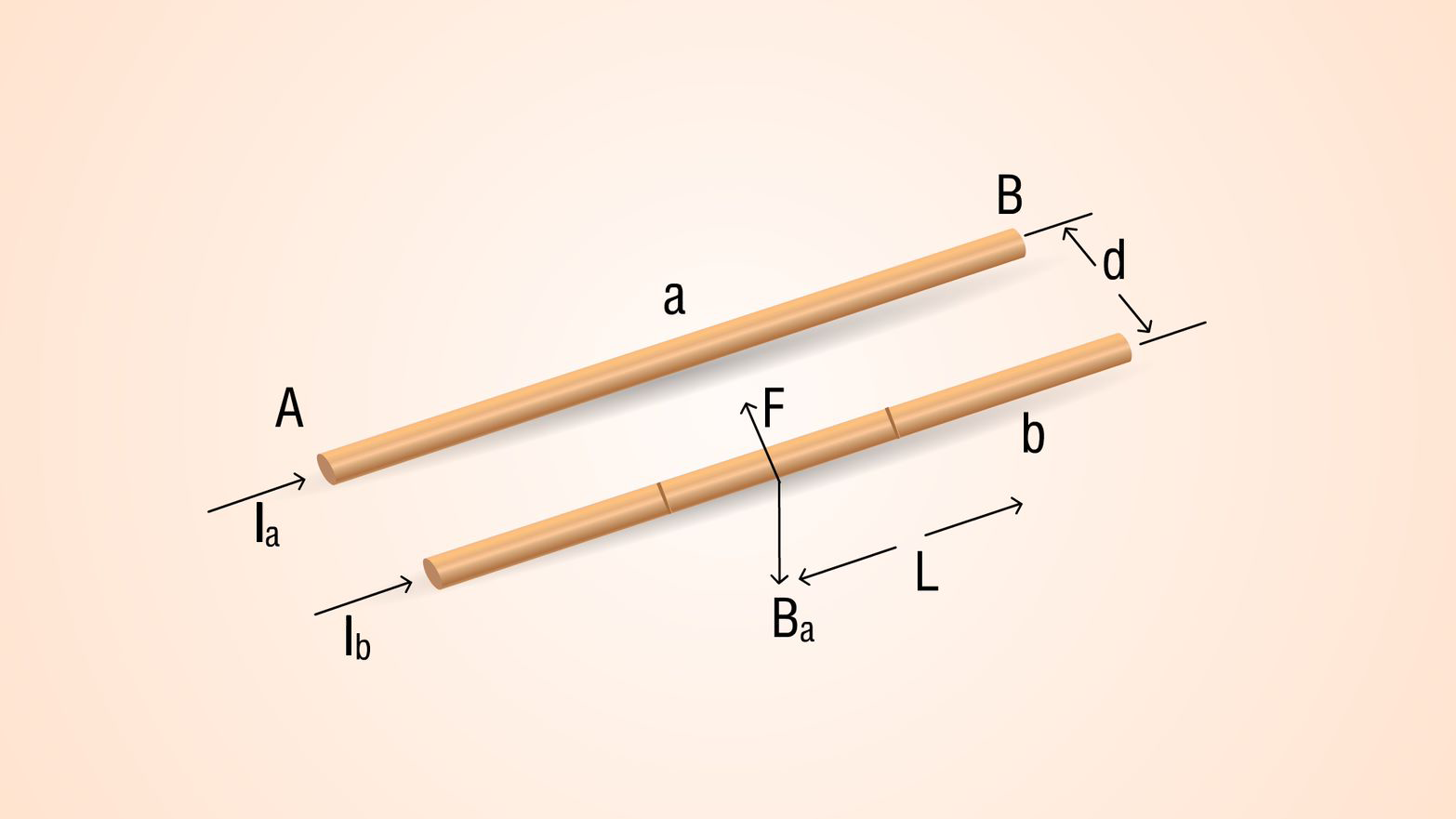Consider two conductors (a and b) are carrying current Ia and Ib. Conductors are placed at a distance of d.

Conductor b experiences a force due to magnetic field of current carrying conductor a.

Magnetic field due to current in conductor a.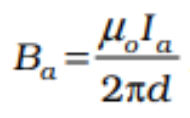µ0 = 4π × 10-7 H/m

We get direction of magnetic field due to current Ia acting on conductor b is downward when we apply right hand thumb rule.

Force on b due to magnetic field Ba.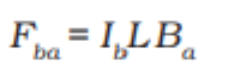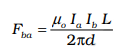• Applying right hand rule for cross products for L and Ba direction of Fbais towards conductor a.
• Force on a due to magnetic field of current carrying conductor b (Fab)= - Fba
• Fba is towards conductor b.
• Fba and Fab attractive forces.

## Currents in opposite direction

Consider two conductors (a and b) are carrying current Ia and Ib. Conductors are placed at a distance of d. Ia and Ib are in opposite directions.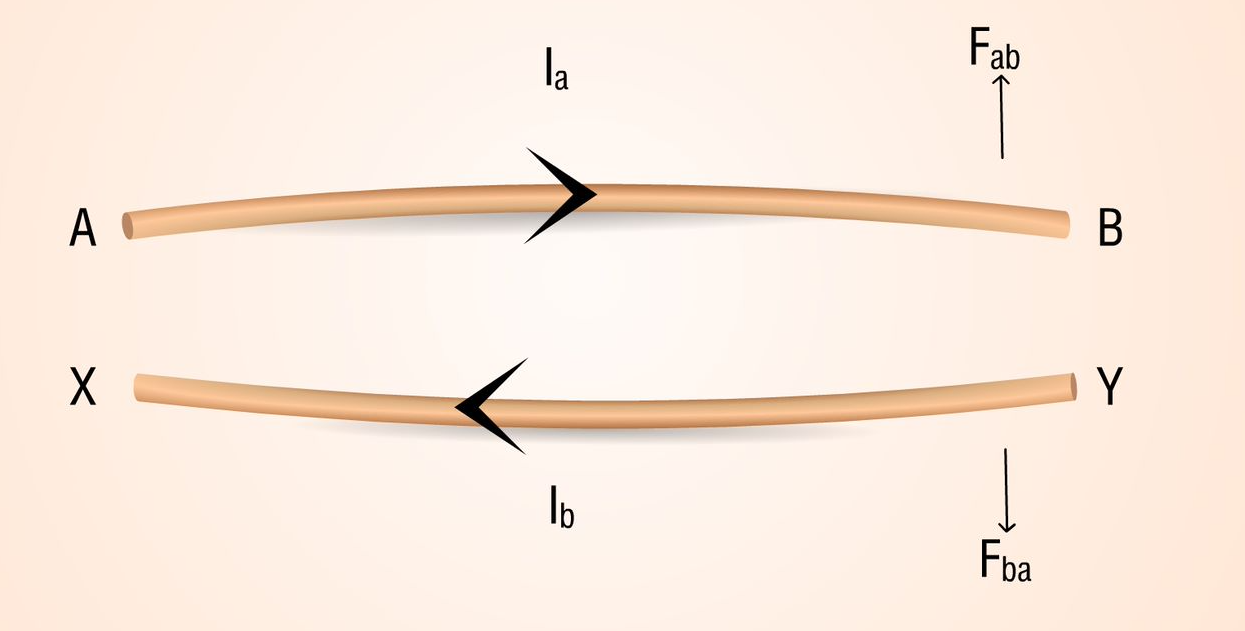• Force on conductor b due to magnetic field of current in conductor a is in direction away from conductor a.
• Force on conductor a due to magnetic field of current in conductor b is in direction away from conductor b.
• Here Fab and Fba are repulsive forces.

## Right hand rule for cross products

a and b are two vectors. When we sweep our fingers from the direction of a to direction of b, direction of thumb gives direction of cross product (a x b).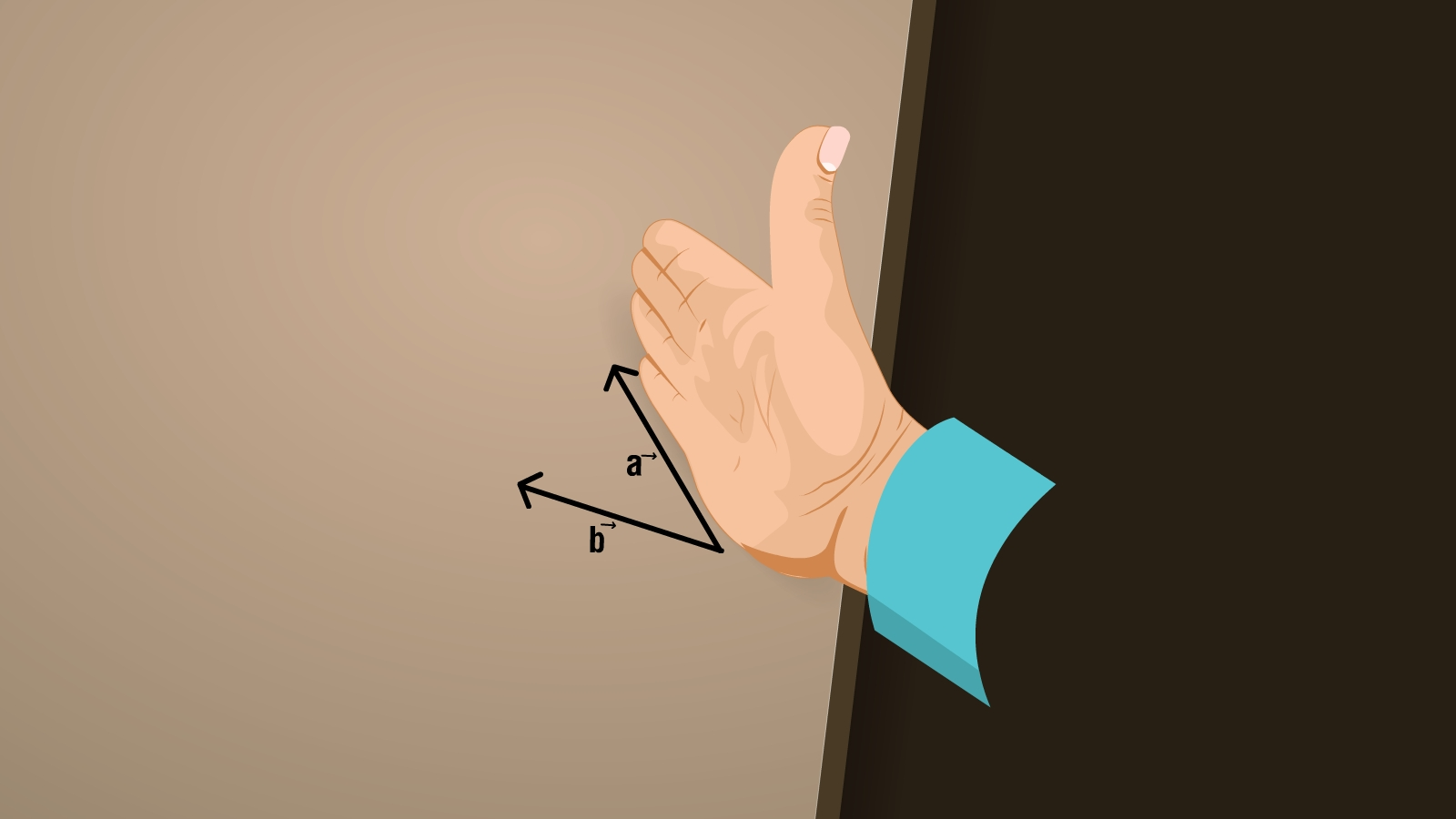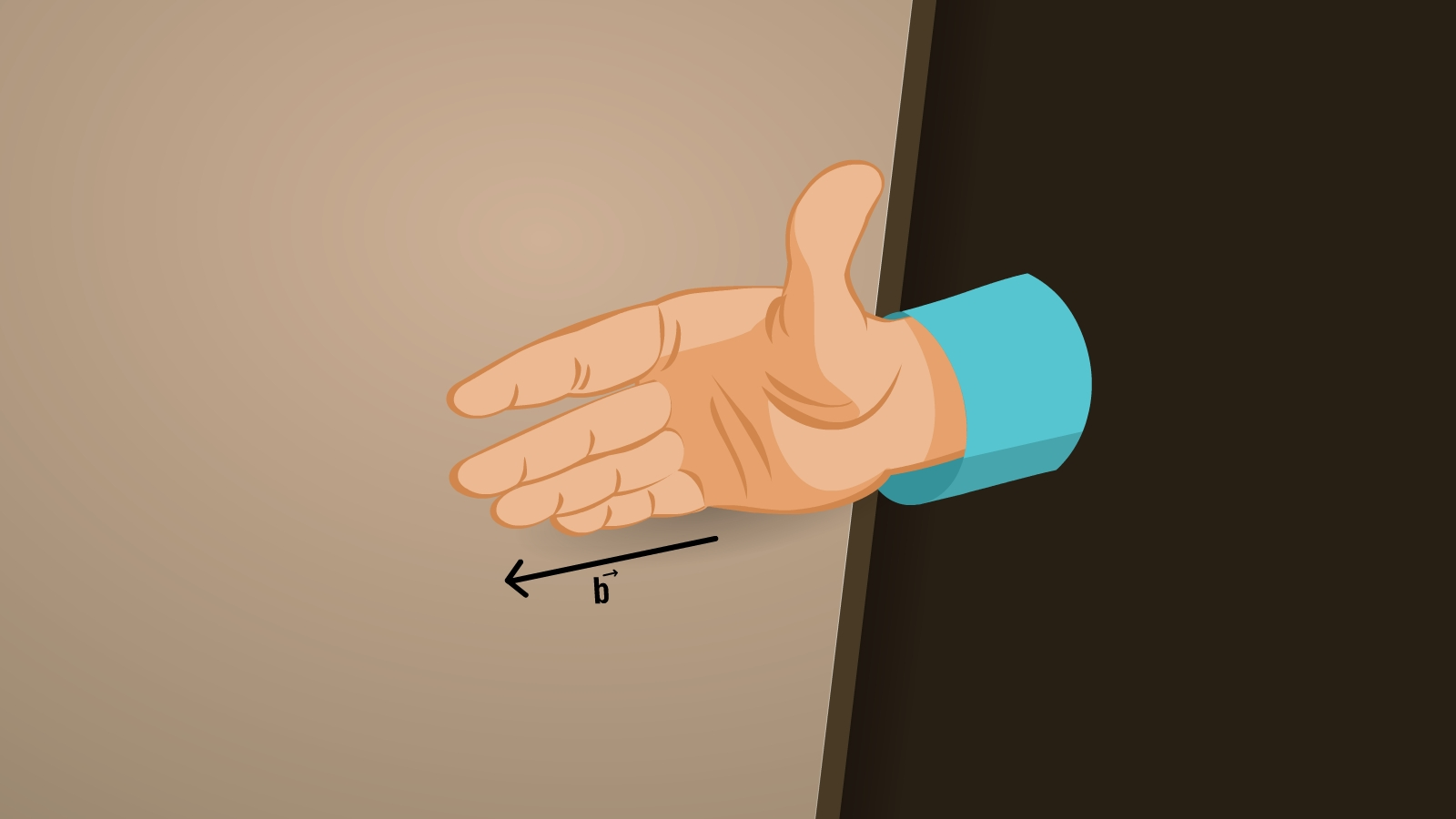## Right hand thumb rule

Curl the palm of your right hand around the circular wire with the fingers pointing in the direction of the current. The right-hand thumb gives the direction of the magnetic field.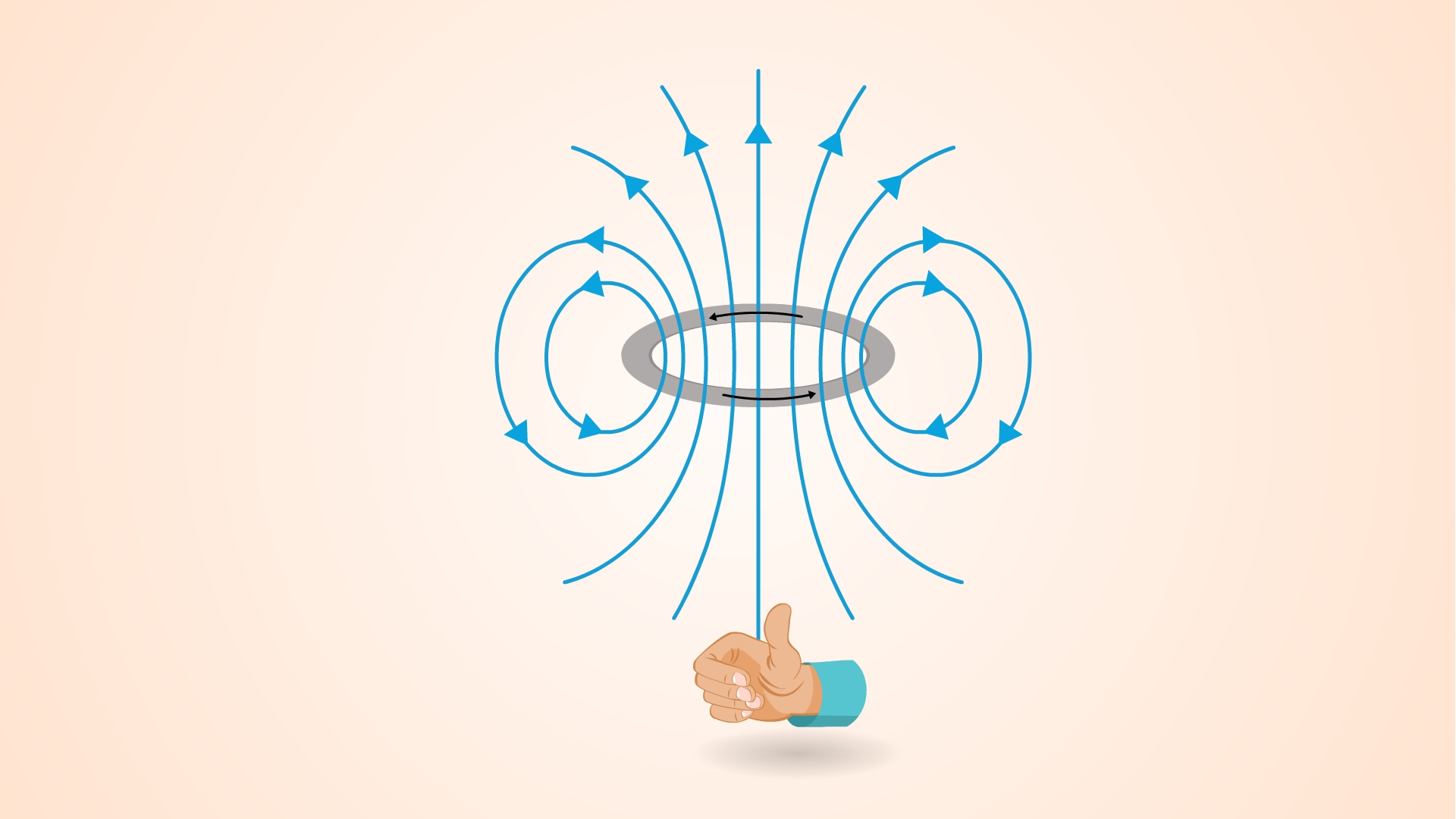# Learning Outcomes

• Students can understand the concept of parallel and antiparallel currents.
• Students can be able to find the direction of force due to magnetic field and direction of magnetic field.